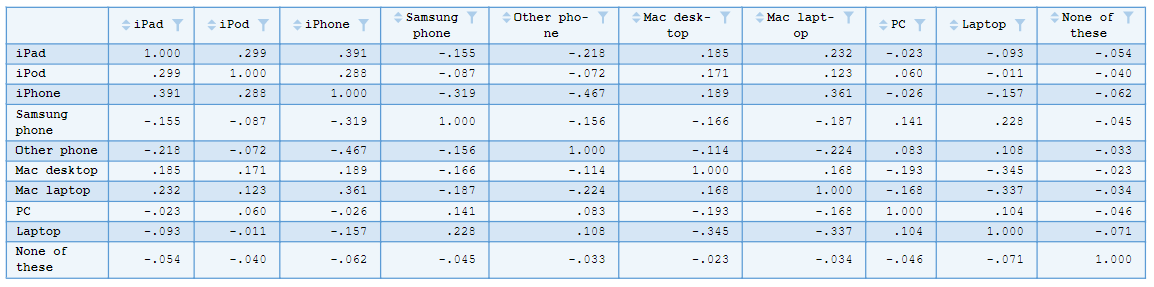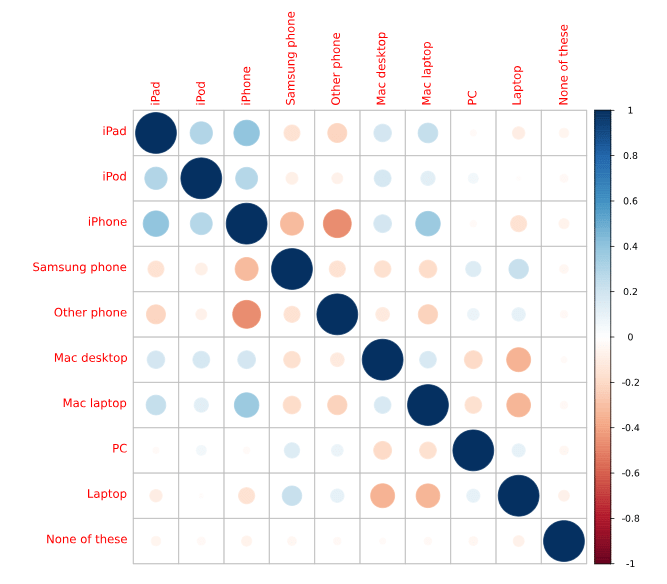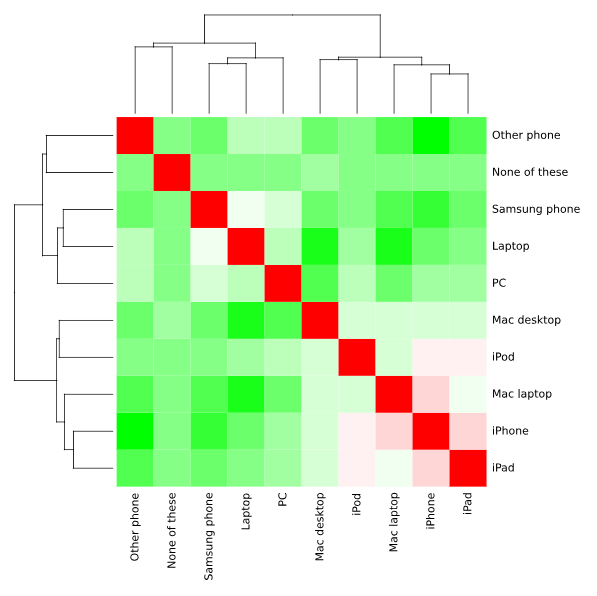Want to share your content on R-bloggers? click here if you have a blog, or here if you don't.

A correlation matrix is a table of correlation coefficients for a set of variables used to determine if a relationship exists between the variables. The coefficient indicates both the strength of the relationship as well as the direction (positive vs. negative correlations). In this post I show you how to calculate and visualize a correlation matrix using R.

As an example, let’s look at a technology survey in which respondents were asked which devices they owned. We want to examine if there is a relationship between any of the devices owned by running a correlation matrix for the device ownership variables. To do this in R, we first load the data into our session using the read.csv function:

```mydata = read.csv("https://wiki.q-researchsoftware.com/images/b/b9/Ownership.csv", header = TRUE, fileEncoding="latin1")
```

## The cor function

The simplest and most straight-forward to run a correlation in R is with the cor function:

```mydata.cor = cor(mydata)
```

This returns a simple correlation matrix showing the correlations between pairs of variables (devices).You can choose the correlation coefficient to be computed using the method parameter. The default method is Pearson, but you can also compute Spearman or Kendall coefficients.

```mydata.cor = cor(mydata, method = c("spearman"))
```

Significance levels (p-values) can also be generated using the rcorr function which is found in the Hmisc package. First install the required package and load the library.

```install.packages("Hmisc")
library("Hmisc")
```

Use the following code to run the correlation matrix with p-values. Note that the data has to be fed to the rcorr function as a matrix.

```mydata.rcorr = rcorr(as.matrix(mydata))
mydata.rcorr
```

This generates one table of correlation coefficients (the correlation matrix) and another table of the p-values. By default, the correlations and p-values are stored in an object of class type rcorr. To extract the values from this object into a useable data structure, you can use the following syntax:

```mydata.coeff = mydata.rcorr\$r
mydata.p = mydata.rcorr\$P
```Objects of class type matrix are generated containing the correlation coefficients and p-values.

## Visualizing the correlation matrix

There are several packages available for visualizing a correlation matrix in R. One of the most common is the corrplot function. We first need to install the corrplot package and load the library.

```install.packages("corrplot")
library(corrplot)
```

Next, we’ll run the corrplot function providing our original correlation matrix as the data input to the function.

```corrplot(mydata.cor)
```

A default correlation matrix plot (called a Correlogram) is generated. Positive correlations are displayed in a blue scale while negative correlations are displayed in a red scale.We can also generate a Heatmap object again using our correlation coefficients as input to the Heatmap. Because the default Heatmap color scheme is quite unsightly, we can first specify a color palette to use in the Heatmap. The value at the end of the function specifies the amount of variation in the color scale. Typically no more than 20 is needed here. We then use the heatmap function to create the output:

```palette = colorRampPalette(c("green", "white", "red")) (20)
heatmap(x = mydata.cor, col = palette, symm = TRUE)
```Want to discover how to do more in R?